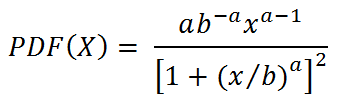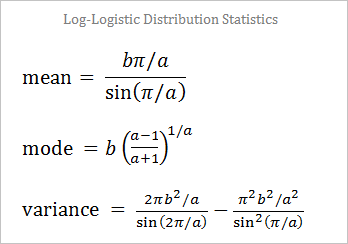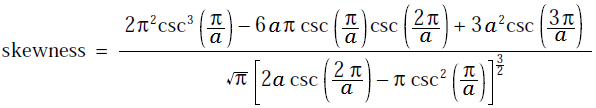# Log-Logistic Random Variable Generator

The log-logistic distribution, also known as the Fisk distribution in economics, is a two-parameter probability distribution. The parameter a governs the shape, while b is a scaling factor. If a random variable X has a logistic distribution, then ln(X) has a log-logistic distribution. The Fisk probability model has a wide variety of applications, including, hydrology, survival analysis, and wealth distribution. You can use the calculator below to generate random numbers from a log-logistic distribution.

 a =       b =       N = Decimal Precision integer tenths hundredths thousandths Display comma separated vertical

The log-logistic probability density function isThe median of the Fisk distribution is b. The mean, mode, variance, and skewness are more complicated functions of a and b:The first and third quartiles of the log-logistic distribution are b(1/3)1/a and b(3)1/a respectively.